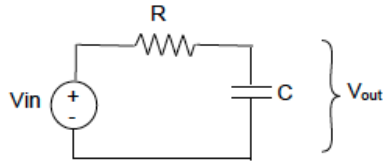## Differential Equations & Filters

The below diagram is an RC circuit being used as a low-pass filter in order to filter a PWM signal generated by a microcontroller. Use Kirchhoff’s voltage law to find the first-order differential equation that relates the voltage across the capacitor (Vout) to Vin, R, and C.Hint
KVL:
$$V_{in}-Ri-V_{out}=0$$$where $$V$$ is the voltage, $$i$$ is the current, and $$R$$ is the resistance. Hint 2 Ohm's Law: $$V=IR$$$
where $$V$$ is the voltage, $$I$$ is the current, and $$R$$ is the resistance.
Using KVL we have:
$$V_{in}-Ri-V_{out}=0$$$where $$V$$ is the voltage, $$i$$ is the current, and $$R$$ is the resistance. We also know that for a capacitor we have: $$C\frac{dv}{dt}=i$$$
Applying Ohm’s Law:
$$V=IR=RC\frac{dV_{out}}{dt}$$$Since the current throughout the circuit must be the same, we can write KVL as: $$V_{in}-RC\frac{dV_{out}}{dt}-V_{out}=0$$$
$$V_{out}=V_{in}-RC\frac{dV_{out}}{dt}$$$$$V_{out}=V_{in}-RC\frac{dV_{out}}{dt}$$$